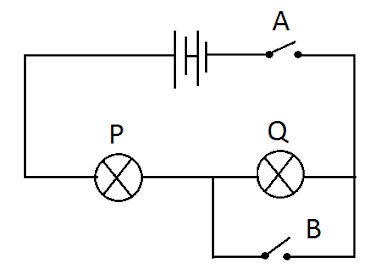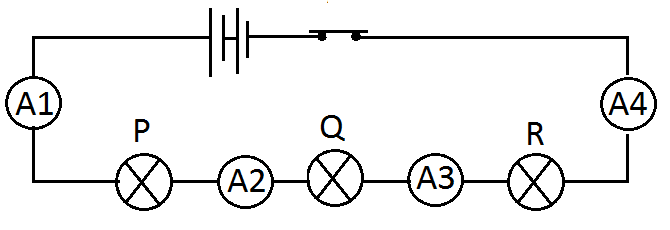Electricity - Circuit problems
10 MCQs on circuit problems
Class *
Study the circuit given below and answer Q1 to Q3Q1 What will you observe if switch A is closed and switch B is kept open? *
1 point
Q2. What will you observe if switch A is open and B is kept closed? *
1 point
Q3. What will you observe if both switches A and B are kept closed? *
1 point
Study the circuit given below and answer Q4Q4. In the above circuit, the relation between the readings of ammeter A1, A2, A3 and A4 is *
1 point